## LetsPlayMaths.Com

WELCOME TO THE WORLD OF MATHEMATICS

# Class 5 Fractions

Multiplication of Fractions

Multiplication of Mixed Fraction With Whole Number

Multiplication of Mixed Fraction With Fraction

Multiplication of Mixed Fractions

Fraction of a Whole Number

Product of More Fractions

Properties of Fraction Multiplication

Reciprocal of Fraction

Division of Fractions

Division of Whole Number by Fraction

Properties of Fraction Division

Fractions Test

Fractions Worksheet

## Multiplication of Fractions

We know multiplication is nothing but repetitive addition, it implies to both whole numbers and fractions.
In case of whole numbers, 4 x 5 = 4 + 4 + 4 + 4 + 4 = 20
In case of fractions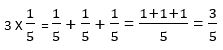Simple way to multiply fractions is multiply numerator with the whole number and leave denominator as it is.

Example 1. Multiply 5 x 35.

Solution.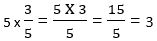Example 2. Multiply 2 x 57.

Solution.## Multiplication of Mixed Fraction With Whole Number

Multiplication of mixed fraction with whole number can be done by two ways.

2. Converting mixed fraction into improper fraction

Example 1. Multiply 335 by 5.

Solution.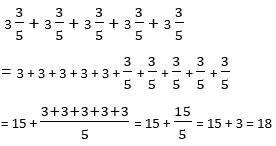Converting mixed fraction into improper fraction

Example 1. Multiply 235 X 4

Solution. First convert the mixed fraction into improper fractionThen multiply 135 by 4## Multiplication of Mixed Fraction With Fraction

In this type of multiplication, we should convert the mixed fraction into improper fraction. Then, we must multiply numerators of both the fractions and keep the result in numerator of the result. After that, denominators should be multiplied, and result should be kept in denominator of the result.

Example 1. Multiply 235 by 34.

Solution.Example 2. Multiply 315 by 58.

Solution.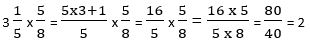## Multiplication of Mixed Fractions

When we multiply two mixed fractions, then we must convert both the mixed fractions into improper fraction and then multiply numerator with numerator and denominator with denominator of the improper fraction.

Example 1. Multiply 215 by 1211.

Solution.Convert the mixed fractions to improper fraction.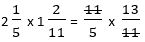11 is present in both numerator and denominator, we can cancel them out as shown above## Fraction of a Whole Number

Fraction of a whole number is similar to the product of fraction with a whole number. Let's have a look at some examples.

Example 1. Number of students in a class is 60. If girl's strength is 13 of the total number of students, then what is the number of girl students?

Solution.Number of girls present in the class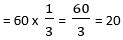So, total number of girl student is 20.

Example 2. Population of a city is 25545. If 15 th of the population are senior citizens, then what is the number of senior citizens leaving in that city?

Solution.Number of senior citizens leaving in the city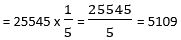## Product of More Fractions

In this type of multiplication, we multiply more than two fractions. Let's have a look at some examples.

Example 1. Find the product of 35, 73 and 27.

Solution.First find the product of any two fractions.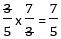Then multiply remaining fraction with 75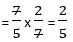Thus, the product of 35, 73 and 27 is equal to 25.

Example 2. Find the product of 45, 73, 57 and 2515.

Solution. First convert mixed fraction into improper fraction and then do the multiplication.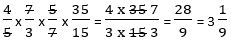Thus, the result is 319.

## Properties of Fraction Multiplication

1. The product of a fraction and zero is zero.

2. The product of a fraction and 1 is the fraction itself.

3. Changing the order of multiplication of the fractions does not change the result.

4. Changing the grouping in the multiplication of three or more fractions does not change the result.

## Reciprocal of Fraction

If the numerator and denominator of a fraction are interchanged, we get the reciprocal of the fraction.

Example 1. Find out the reciprocal of 35.

Solution. Here numerator is 3 and denominator 5. By interchanging the numerator and the denominator, the reciprocal of 35 is 53.

## Division of Fractions

Division of fraction can be performed by following below mentioned steps.

1. Find the reciprocal of the divisor by interchanging the numerator and denominator.

2. Multiply the dividend with the reciprocal of the divisor.

Example 1. Divide 35 by 15.

Solution. Reciprocal of 15 is 5.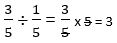Thus, the result is 3.

Example 2. Divide 311 by 11.

Solution. Reciprocal of 11 is 111.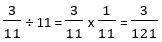Example 3. Divide 512 by 213.

Solution. Convert the mixed fractions into improper fractions.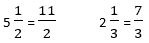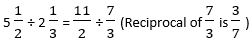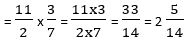## Division of Whole Number by Fraction

To divide a whole number by a fraction, we must multiply the reciprocal of the fraction with the whole number. Let's have a look at some examples.

Example 1. Divide 8 by 23.

Solution. Reciprocal of 23 is 32.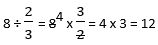Example 2. Divide 9 by 112.

Solution. Convert the mixed fraction into improper fraction = 112 = 32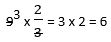Reciprocal of 32 is 23.

## Properties of Fraction Division

1. When zero is divided by a fraction, then the result will be zero.

2. When fraction is divided by 1, then the result will be the fraction itself.

3. When 1 is divided by a fraction, then the result will be the reciprocal of the fraction.

4. When a fraction is divided by itself, then the result will be one.

## Fractions Test

Fractions Test - 1

Fractions Test - 2

## Class-5 Fractions Worksheet

Fractions Worksheet - 1

Fractions Worksheet - 2

Fractions Worksheet - 3

Fractions Worksheet - 4

Fractions Worksheet - 5

Fractions Worksheet - 6

Fractions Worksheet - 7